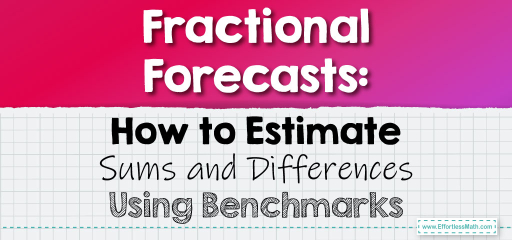# Fractional Forecasts: How to Estimate Sums and Differences Using Benchmarks

When working with fractions, sometimes an exact calculation isn't necessary. Instead, a close estimate will suffice. Benchmarks, such as $$0$$, $$1/2$$, and $$1$$, can be invaluable tools for quickly estimating the sum or difference of fractions. Let's explore how to use these benchmarks for estimating operations with fractions.## Estimating Sums and Differences of Fractions Using Benchmarks

### Example 1:

Estimate the sum of $$2/3$$ and $$3/8$$.

Estimation Process Using Benchmarks:

1. $$2/3$$ is closer to $$1$$ than $$1/2$$, so round it to $$1$$.

2. $$3/8$$ is closer to $$1/2$$ than $$0$$, so round it to $$1/2$$.

3. Estimate the sum: $$1 + 1/2 = 1 1/2$$.

The estimated sum is $$1 1/2$$.

The Absolute Best Book for 5th Grade Students

### Example 2:

Estimate the difference between $$5/6$$ and $$1/4$$.

Estimation Process Using Benchmarks:

1. $$5/6$$ is closer to $$1$$ than $$1/2$$, so round it to $$1$$.

2. $$1/4$$ is closer to $$0$$ than $$1/2$$, so round it to $$0$$.

3. Estimate the difference: $$1 – 0 = 1$$.

The estimated difference is $$1$$.

Using benchmarks to estimate sums and differences of fractions provides a quick and intuitive approach to understanding the approximate outcome of operations. This method is especially useful when you need a general idea rather than precise calculations. By rounding fractions to the nearest benchmark and then performing the operation, you can get a ballpark figure swiftly. Practice regularly to master this invaluable skill!

### Practice Questions:

1. Estimate the sum of $$4/5$$ and $$3/7$$.

2. What is the estimated difference between $$7/8$$ and $$2/3$$?

3. Using benchmarks, estimate the sum of $$1/3$$ and $$5/9$$.

4. Estimate the difference between $$3/4$$ and $$1/6$$.

5. What is the estimated sum of $$2/7$$ and $$4/5$$?

A Perfect Book for Grade 5 Math Word Problems!

1. $$1 1/2$$

2. $$1/4$$

3. $$1$$

4. $$1/2$$

5. $$1 1/4$$

The Best Math Books for Elementary Students

### What people say about "Fractional Forecasts: How to Estimate Sums and Differences Using Benchmarks - Effortless Math: We Help Students Learn to LOVE Mathematics"?

No one replied yet.

X
51% OFF

Limited time only!

Save Over 51%

SAVE $15 It was$29.99 now it is \$14.99